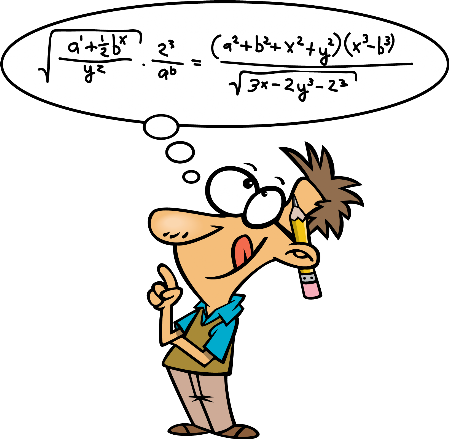# Costing Equation Transcript - BC Bookkeeping Tutorials|dwmbeancounter.comTitle
Go to content

## Costing Equation Transcript

Cost Behaviors > Costing EquationThe cost equation is a mathematical formula used to predict costs at various levels of volume.

The cost equation is Y = (V *X) + F.
Y is the total mix costs. V is the variable cost per unit,X is the volume, and F is the total fixed costs.

Another way to think of the cost equation is as follows; total cost equals variable cost per unit times volume plus fixed costs.

The cost equation is only valid within a relevant range. Outside the relevant range of volume the cost equation changes.

For example, if I owned one airplane I could predict costs at various levels of volume for flights from Salt Lake to Las Vegas. I could determine how many passengers I could fly and how many flights I could make in a day. All of those things are within the relevant range, however once demand got so large that I needed a second airplane to meet the demand, the relevant range changes and the original cost equation is no longer valid.

In this case the fixed costs would go way up when I had to buy a second aircraft.

Graphically you can see how the costs change over time.

Total variable costs are often curvilinear, which means they increase but not always in proportion to the volume at any level of volume.

Think about the purchasing power of Wal-Mart vs. a local store. As Wal-Mart gets larger and larger they can get discounts at volume levels that many smaller stores cannot.
Fixed costs are fixed until volume increases too much that a new production plant or airplanes have to be placed in service.

Fixed costs tend to be a step costs as volume increases.

So our ability to predict costs using the cost equation is only valid when we are within the relevant range of volume.

Back to content